# Question 1.Use the given letters to write the answer.i. There are ‘a’ trees in the village Lat. If the number of trees increases every year by ‘b’, then how many trees will there be after ‘x’ years?ii. For the parade there are y students in each row and x such row is formed. Then, how many students are there for the parade in all?iii. The tens and units place of a two-digit number is m and n respectively. Write the polynomial which represents the two-digit number.Answer:i. Given:Total number of trees in the village = aIncrease in the number of trees by = bTo find: Number of trees after x yearsNumber of trees in x years = (a + b) xii. Given:Number of students in each row = yNumber of rows of Students = xSo total number of students = x × y= xyiii. For a number in tens place should be multiplied with ten and for the number in units place should be multiplied by one. So the number that can be formed as follows:Since m is in tens place and n is in units place,10 × m + 1 × n10m + nSo the polynomial representing the two digit number is = 10m + nQuestion 2.Add the given polynomials.i. x3 – 2x2 – 9; 5x3 + 2x +9ii.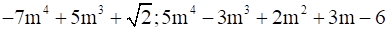iii. 2y2 + 5; 3y +9; 3y2 – 4y – 3Answer:i.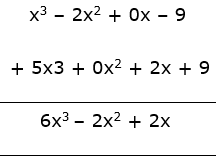The result = 6x3 – 2x2 + 2xii.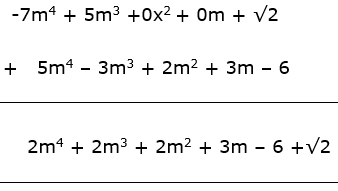The result = 2m4 + 2m3 + 2m2 + 3m – 6 +√2iii.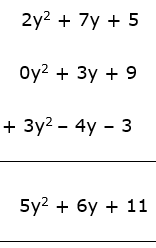The result = 5y2 + 6y + 11Question 3.Subtract the second polynomial from the first.i.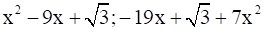ii. 2ab2 + 3a2b – 4ab; 3ab – 8ab2 +2a2bAnswer:In the subtraction process, the sign of the subtrahend that is the second polynomial is inverted and then the operation is carried out.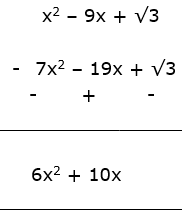The result = 6x2 + 10xii. In the subtraction process, the sign of the subtrahend that is the second polynomial is inverted and then the operation is carried out.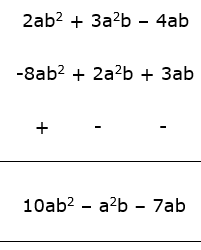The result = 10ab2 – a2b – 7abQuestion 4.Multiply the given polynomials.i. 2x; x2 – 2xii. x5 – 1; x3 + 2x3 + 2iii. 2y + 1; y2 – 2y3 + 3yAnswer:The multiplication is as follows:⇒ 2x × (x2 – 2x – 1)⇒ 2x.x2 – 2x.2x – 2x.1⇒ 2x2+1 – 4x1+1 – 2x⇒ 2x3 – 4x2 – 2xTherefore, the product = 2x3 – 4x2 – 2x“.” represents multiplicationii. The multiplication is as follows:⇒ (x5 – 1) × (x3 + 2x3 + 2)⇒ x5.x3 + 2x3.x5 + 2.x5 – 1.x3 – 1.2x3 – 1.2⇒ x5+3 + 2x3 + 5 +2x5 – x3 – 2x3 – 2⇒ x8 + 2x8 + 5 + 2x5 – x3 – 2x3 – 2⇒ 3x8 + 2x5 + 3x3 + 3The product is = 3x8 + 2x5 + 3x3 + 3“.” represents multiplicationiii. The multiplication is as follows:⇒ (2y + 1) × (y2 – 2y3 + 3y)⇒ 2y.y2 – 2y3.2y + 3y.2y + 1.y2 – 1.2y3 + 1.3y⇒ 2y2+1 – 4y3+1 + 6y1+1 + y2 – 2y3 + 3y⇒ 2y3 – 4y4 + 6y2 + y2 – 2y3 + 3y⇒ -4y4 + 7y2 + 3yThe product is = -4y4 + 7y2 + 3y“.” represents multiplicationQuestion 5.Divide first polynomial by second polynomial and write the answer in the form ‘Dividend = Divisor × Quotient + Remainder’.i. x3 – 64; x – 4ii. 5x5 + 4x4 – 3x3 + 2x2 + 2; x2 – xAnswer:i. The division is as follows: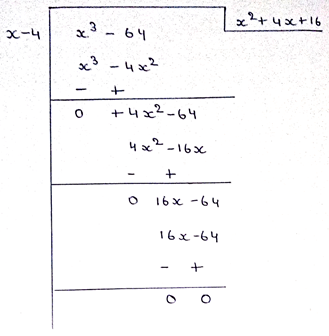x3-64 = [(x2 + 4x + 16) × (x-4)] + 0x3-64 = (x2 + 4x + 16) × (x-4)ii. The division is as follows: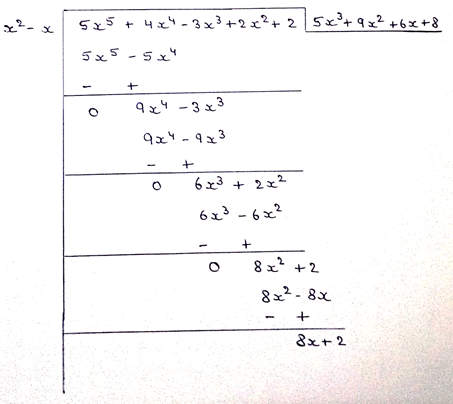5x5 + 4x4 – 3x3 + 2x2 + 2 = [(5x3 + 9x2 + 6x + 8) × (x2-x)] + 8x +2Quotient = 5x3 + 9x2 + 6x + 8Remainder = 8x + 2Question 6.Write down the information in the form of algebraic expression and simplify.There is a rectangular farm with length (2a2 + 3b2) meter and breadth (a2 + b2) meter. The farmer used a square shaped plot of the farm to build a house. The side of the plot was (a2 - b2) meter. What is the area of the remaining part of the farm?Answer:Given:Length of the farm, l = (2a2 + 3b2) mBreadth of the farm, b = (a2 + b2) mSide of the square plot, s = (a2 - b2) mTo find: Area of remaining farmExplain:Area of Remaining Farm = Area of Rectangle – Area of SquareArea of Rectangle = l × b= (2a2 + 3b2) × (a2 + b2)= 2a2.a2 + 2a2.b2 + 3b2.a2 + 3b2.b2= 2a4 + 5a2b2 + 3b4Area of Square = s × s= (a2 - b2) × (a2 - b2)= a2.a2 – a2.b2 – a2.b2 + b2.b2= a4 – 2a2b2 + b4Area of Remaining Part = (2a4 + 5a2b2 + 3b4) – (a4 – 2a2b2 + b4)= 2a4 + 5a2b2 + 3b4 - a4 + 2a2b2 - b4= a4 + 7a2b2 + 2b4Therefore, the area of remaining portion is = a4 + 7a2b2 + 2b4

## PDF FILE TO YOUR EMAIL IMMEDIATELY PURCHASE NOTES & PAPER SOLUTION. @ Rs. 50/- each (GST extra)

SUBJECTS

HINDI ENTIRE PAPER SOLUTION

MARATHI PAPER SOLUTION
SSC MATHS I PAPER SOLUTION
SSC MATHS II PAPER SOLUTION
SSC SCIENCE I PAPER SOLUTION
SSC SCIENCE II PAPER SOLUTION
SSC ENGLISH PAPER SOLUTION
SSC & HSC ENGLISH WRITING SKILL
HSC ACCOUNTS NOTES
HSC OCM NOTES
HSC ECONOMICS NOTES
HSC SECRETARIAL PRACTICE NOTES

2019 Board Paper Solution

HSC ENGLISH SET A 2019 21st February, 2019

HSC ENGLISH SET B 2019 21st February, 2019

HSC ENGLISH SET C 2019 21st February, 2019

HSC ENGLISH SET D 2019 21st February, 2019

SECRETARIAL PRACTICE (S.P) 2019 25th February, 2019

HSC XII PHYSICS 2019 25th February, 2019

CHEMISTRY XII HSC SOLUTION 27th, February, 2019

OCM PAPER SOLUTION 2019 27th, February, 2019

HSC MATHS PAPER SOLUTION COMMERCE, 2nd March, 2019

HSC MATHS PAPER SOLUTION SCIENCE 2nd, March, 2019

SSC ENGLISH STD 10 5TH MARCH, 2019.

HSC XII ACCOUNTS 2019 6th March, 2019

HSC XII BIOLOGY 2019 6TH March, 2019

HSC XII ECONOMICS 9Th March 2019

SSC Maths I March 2019 Solution 10th Standard11th, March, 2019

SSC MATHS II MARCH 2019 SOLUTION 10TH STD.13th March, 2019

SSC SCIENCE I MARCH 2019 SOLUTION 10TH STD. 15th March, 2019.

SSC SCIENCE II MARCH 2019 SOLUTION 10TH STD. 18th March, 2019.

SSC SOCIAL SCIENCE I MARCH 2019 SOLUTION20th March, 2019

SSC SOCIAL SCIENCE II MARCH 2019 SOLUTION, 22nd March, 2019

XII CBSE - BOARD - MARCH - 2019 ENGLISH - QP + SOLUTIONS, 2nd March, 2019

HSC Maharashtra Board Papers 2020

(Std 12th English Medium)

HSC ECONOMICS MARCH 2020

HSC OCM MARCH 2020

HSC ACCOUNTS MARCH 2020

HSC S.P. MARCH 2020

HSC ENGLISH MARCH 2020

HSC HINDI MARCH 2020

HSC MARATHI MARCH 2020

HSC MATHS MARCH 2020

SSC Maharashtra Board Papers 2020

(Std 10th English Medium)

English MARCH 2020

HindI MARCH 2020

Hindi (Composite) MARCH 2020

Marathi MARCH 2020

Mathematics (Paper 1) MARCH 2020

Mathematics (Paper 2) MARCH 2020

Sanskrit MARCH 2020

Important-formula

THANKS